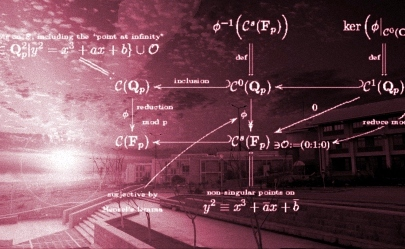# Dersler

Katalog İçerikleri

MAT 100 Precalculus (1-2)2
Mat 100 is a preparatory course for calculus courses. Topics include: Functions and their inverses, operations with functions and graphing techniques, polynomial functions, rational functions, exponential and logarithmic functions, trigonometric functions, trigonometric identities and trigonometric equations, systems of equations, inequalities and solving techniques.

MAT 119 Calculus with Analytic Geometry (4-2)5
Functions, limits, continuity and derivatives. Applications. Extreme values, the Mean Value Theorem and its applications. Graphing. The definite integral. Area and volume as integrals. The indefinite integral. Transcendental functions and their derivatives. L`Hospital`s rule. Techniques of integration. Improper integrals. Applications.

MAT 120 Calculus for Functions of Several Variables (4-2)5
Sequences, infinite series, power series, Taylor series. Vectors, lines and planes in space. Functions of several variables: Limit, continuity, partial derivatives, the chain rule, directional derivatives, tangent plane approximation and differentials extreme values, Lagrange multipliers. Double integrals with applications. The line integral. Prerequisite: MAT 119.

MAT 210 Applied Mathematics for Engineers (4-0)4
Vector differential and integral calculus. Matrices. Determinant. Systems of linear equations. Characteristic values and characteristic vectors of matrices. Introduction to numerical methods. Prerequisite: MAT 120 or consent of the Department.

MAT 219 Differential Equations (4-0)4
Existence and uniqueness theorems. First order equations. Trajectories. Higher order linear equations; undetermined coefficients, variation of parameters and operator methods. Power series solutions. Laplace transform solutions of IVP's. Theory of linear systems. Solutions by operator, Laplace and linear algebra methods. Partial differential equations, separation of variables and Fourier series. Prerequisites: MAT 119 and MAT 120.

MAT 260 Basic Linear Algebra, (3-0)3
Matrices, determinants and systems of linear equations. Vector spaces, the Euclidian space, inner product spaces, linear transformations. Eigenvalues, diagonalization.